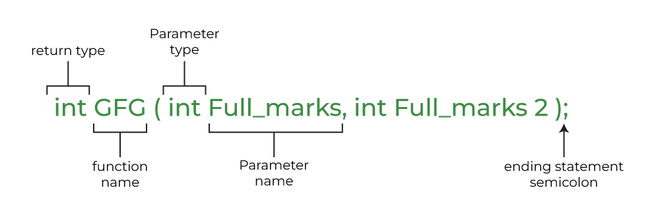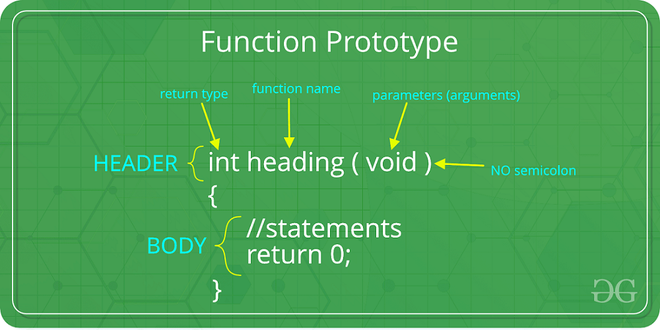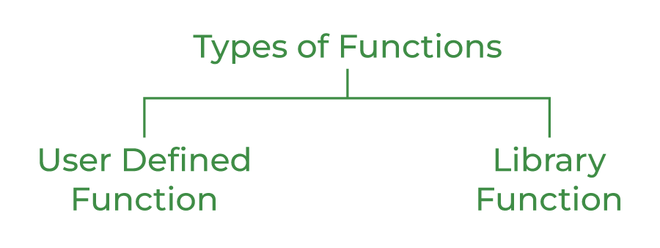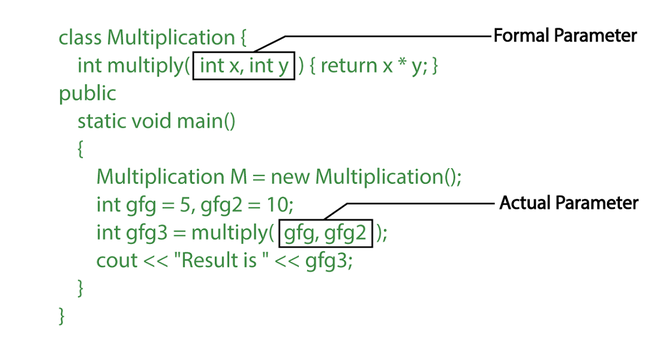GFG App
Open AppBrowser
Continue

# Functions in C++

A function is a set of statements that take inputs, do some specific computation, and produce output. The idea is to put some commonly or repeatedly done tasks together and make a function so that instead of writing the same code again and again for different inputs, we can call the function.
In simple terms, a function is a block of code that only runs when it is called.

Syntax:Syntax of Function

Example:

## C++

 `// C++ Program to demonstrate working of a function` `#include ` `using` `namespace` `std;`   `// Following function that takes two parameters 'x' and 'y'` `// as input and returns max of two input numbers` `int` `max(``int` `x, ``int` `y)` `{` `    ``if` `(x > y)` `        ``return` `x;` `    ``else` `        ``return` `y;` `}`   `// main function that doesn't receive any parameter and` `// returns integer` `int` `main()` `{` `    ``int` `a = 10, b = 20;`   `    ``// Calling above function to find max of 'a' and 'b'` `    ``int` `m = max(a, b);`   `    ``cout << ``"m is "` `<< m;` `    ``return` `0;` `}`

Output

`m is 20`

Time complexity: O(1)

Space complexity: O(1)

## Why Do We Need Functions?

• Functions help us in reducing code redundancy. If functionality is performed at multiple places in software, then rather than writing the same code, again and again, we create a function and call it everywhere. This also helps in maintenance as we have to change at one place if we make future changes to the functionality.
• Functions make code modular. Consider a big file having many lines of code. It becomes really simple to read and use the code if the code is divided into functions.
• Functions provide abstraction. For example, we can use library functions without worrying about their internal work.

## Function Declaration

A function declaration tells the compiler about the number of parameters function takes data-types of parameters, and returns the type of function. Putting parameter names in the function declaration is optional in the function declaration, but it is necessary to put them in the definition. Below are an example of function declarations. (parameter names are not there in the below declarations)Function Declaration

Example:

## C++

 `// C++ Program to show function that takes` `// two integers as parameters and returns` `// an integer` `int` `max(``int``, ``int``);`   `// A function that takes an int` `// pointer and an int variable` `// as parameters and returns` `// a pointer of type int` `int``* swap(``int``*, ``int``);`   `// A function that takes` `// a char as parameter and` `// returns a reference variable` `char``* call(``char` `b);`   `// A function that takes a` `// char and an int as parameters` `// and returns an integer` `int` `fun(``char``, ``int``);`

## Types of FunctionsTypes of Function in C++

### User Defined Function

User Defined functions are user/customer-defined blocks of code specially customized to reduce the complexity of big programs. They are also commonly known as “tailor-made functions” which are built only to satisfy the condition in which the user is facing issues meanwhile reducing the complexity of the whole program.

### Library Function

Library functions are also called “builtin Functions“. These functions are a part of a compiler package that is already defined and consists of a special function with special and different meanings. Builtin Function gives us an edge as we can directly use them without defining them whereas in the user-defined function we have to declare and define a function before using them.
For Example: sqrt(), setw(), strcat(), etc.

## Parameter Passing to Functions

The parameters passed to function are called actual parameters. For example, in the program below, 5 and 10 are actual parameters.
The parameters received by the function are called formal parameters. For example, in the above program x and y are formal parameters.Formal Parameter and Actual Parameter

There are two most popular ways to pass parameters:

1. Pass by Value: In this parameter passing method, values of actual parameters are copied to the function’s formal parameters and the two types of parameters are stored in different memory locations. So any changes made inside functions are not reflected in the actual parameters of the caller.

2. Pass by Reference: Both actual and formal parameters refer to the same locations, so any changes made inside the function are actually reflected in the actual parameters of the caller.

## Function Definition

Pass by value is used where the value of x is not modified using the function fun().

## C++

 `// C++ Program to demonstrate function definition` `#include ` `using` `namespace` `std;`   `void` `fun(``int` `x)` `{` `    ``// definition of` `    ``// function` `    ``x = 30;` `}`   `int` `main()` `{` `    ``int` `x = 20;` `    ``fun(x);` `    ``cout << ``"x = "` `<< x;` `    ``return` `0;` `}`

Output

`x = 20`

Time complexity: O(1)

Space complexity: O(1)

## Functions Using Pointers

The function fun() expects a pointer ptr to an integer (or an address of an integer). It modifies the value at the address ptr. The dereference operator * is used to access the value at an address. In the statement ‘*ptr = 30’, the value at address ptr is changed to 30. The address operator & is used to get the address of a variable of any data type. In the function call statement ‘fun(&x)’, the address of x is passed so that x can be modified using its address.

## C++

 `// C++ Program to demonstrate working of` `// function using pointers` `#include ` `using` `namespace` `std;`   `void` `fun(``int``* ptr) { *ptr = 30; }`   `int` `main()` `{` `    ``int` `x = 20;` `    ``fun(&x);` `    ``cout << ``"x = "` `<< x;`   `    ``return` `0;` `}`

Output

`x = 30`

Time complexity: O(1)

Space complexity: O(1)

## Points to Remember About Functions in C++

1. Most C++ program has a function called main() that is called by the operating system when a user runs the program.

2. Every function has a return type. If a function doesn’t return any value, then void is used as a return type. Moreover, if the return type of the function is void, we still can use the return statement in the body of the function definition by not specifying any constant, variable, etc. with it, by only mentioning the ‘return;’ statement which would symbolize the termination of the function as shown below:

## C++

 `void` `function name(``int` `a)` `{` `    ``....... ``// Function Body` `        ``return``; ``// Function execution would get terminated` `}`

3. To declare a function that can only be called without any parameter, we should use “void fun(void)“. As a side note, in C++, an empty list means a function can only be called without any parameter. In C++, both void fun() and void fun(void) are same.

## Main Function

The main function is a special function. Every C++ program must contain a function named main. It serves as the entry point for the program. The computer will start running the code from the beginning of the main function.

## CPP

 `// Without Parameters` `int` `main() { ... ``return` `0; }`

## CPP

 `// With Parameters` `int` `main(``int` `argc, ``char``* ``const` `argv[]) { ... ``return` `0; }`

The reason for having the parameter option for the main function is to allow input from the command line. When you use the main function with parameters, it saves every group of characters (separated by a space) after the program name as elements in an array named argv
Since the main function has the return type of int, the programmer must always have a return statement in the code. The number that is returned is used to inform the calling program what the result of the program’s execution was. Returning 0 signals that there were no problems.

## C++ Recursion

When function is called within the same function, it is known as recursion in C++. The function which calls the same function, is known as recursive function.
A function that calls itself, and doesn’t perform any task after function call, is known as tail recursion. In tail recursion, we generally call the same function with return statement.
Syntax:

## C++

 `recursionfunction()` `{` `    ``recursionfunction(); ``// calling self function` `}`

## C++ Passing Array to Function

In C++, to reuse the array logic, we can create function. To pass array to function in C++,  we need to provide only array name.

`functionname(arrayname); //passing array to function`

Example: Print minimum number

## C++

 `#include ` `using` `namespace` `std;` `void` `printMin(``int` `arr);` `int` `main()` `{` `    ``int` `ar = { 30, 10, 20, 40, 50 };` `    ``printMin(ar); ``// passing array to function` `}` `void` `printMin(``int` `arr)` `{` `    ``int` `min = arr;` `    ``for` `(``int` `i = 0; i < 5; i++) {` `        ``if` `(min > arr[i]) {` `            ``min = arr[i];` `        ``}` `    ``}` `    ``cout << ``"Minimum element is: "` `<< min << ``"\n"``;` `}`   `// Code submitted by Susobhan Akhuli`

Output

```Minimum element is: 10
```

Time complexity: O(n) where n is the size of  array

Space complexity: O(n) where n is the size of array.

If we create two or more members having the same name but different in number or type of parameter, it is known as C++ overloading. In C++, we can overload:

• methods,
• constructors and
• indexed properties

It is because these members have parameters only.

Function Overloading is defined as the process of having two or more function with the same name, but different in parameters is known as function overloading in C++. In function overloading, the function is redefined by using either different types of arguments or a different number of arguments. It is only through these differences compiler can differentiate between the functions.
The advantage of Function overloading is that it increases the readability of the program because you don’t need to use different names for the same action.
Example: changing number of arguments of add() method

## C++

 `// program of function overloading when number of arguments` `// vary` `#include ` `using` `namespace` `std;` `class` `Cal {` `public``:` `    ``static` `int` `add(``int` `a, ``int` `b) { ``return` `a + b; }` `    ``static` `int` `add(``int` `a, ``int` `b, ``int` `c)` `    ``{` `        ``return` `a + b + c;` `    ``}` `};` `int` `main(``void``)` `{` `    ``Cal C; ``// class object declaration.` `    ``cout << C.add(10, 20) << endl;` `    ``cout << C.add(12, 20, 23);` `    ``return` `0;` `}`   `// Code Submitted By Susobhan Akhuli`

Output

```30
55```

Time complexity: O(1)

Space complexity: O(1)

Example: when the type of the arguments vary.

## C++

 `// Program of function overloading with different types of` `// arguments.` `#include ` `using` `namespace` `std;` `int` `mul(``int``, ``int``);` `float` `mul(``float``, ``int``);`   `int` `mul(``int` `a, ``int` `b) { ``return` `a * b; }` `float` `mul(``double` `x, ``int` `y) { ``return` `x * y; }` `int` `main()` `{` `    ``int` `r1 = mul(6, 7);` `    ``float` `r2 = mul(0.2, 3);` `    ``cout << ``"r1 is : "` `<< r1 << endl;` `    ``cout << ``"r2 is : "` `<< r2 << endl;` `    ``return` `0;` `}`   `// Code Submitted By Susobhan Akhuli`

Output

```r1 is : 42
r2 is : 0.6
```

Time Complexity: O(1)

Space Complexity: O(1)

When the compiler is unable to decide which function is to be invoked among the overloaded function, this situation is known as function overloading.
When the compiler shows the ambiguity error, the compiler does not run the program.

• Type Conversion.
• Function with default arguments.
• Function with pass by reference.

Type Conversion:-

## C++

 `#include ` `using` `namespace` `std;` `void` `fun(``int``);` `void` `fun(``float``);` `void` `fun(``int` `i) { cout << ``"Value of i is : "` `<< i << endl; }` `void` `fun(``float` `j)` `{` `    ``cout << ``"Value of j is : "` `<< j << endl;` `}` `int` `main()` `{` `    ``fun(12);` `    ``fun(1.2);` `    ``return` `0;` `}`   `// Code Submitted By Susobhan Akhuli`

The above example shows an error “call of overloaded ‘fun(double)’ is ambiguous“. The fun(10) will call the first function. The fun(1.2) calls the second function according to our prediction. But, this does not refer to any function as in C++, all the floating point constants are treated as double not as a float. If we replace float to double, the program works. Therefore, this is a type conversion from float to double.
Function with Default Arguments:-

## C++

 `#include ` `using` `namespace` `std;` `void` `fun(``int``);` `void` `fun(``int``, ``int``);` `void` `fun(``int` `i) { cout << ``"Value of i is : "` `<< i << endl; }` `void` `fun(``int` `a, ``int` `b = 9)` `{` `    ``cout << ``"Value of a is : "` `<< a << endl;` `    ``cout << ``"Value of b is : "` `<< b << endl;` `}` `int` `main()` `{` `    ``fun(12);`   `    ``return` `0;` `}`   `// Code Submitted By Susobhan Akhuli`

The above example shows an error “call of overloaded ‘fun(int)’ is ambiguous“. The fun(int a, int b=9) can be called in two ways: first is by calling the function with one argument, i.e., fun(12) and another way is calling the function with two arguments, i.e., fun(4,5). The fun(int i) function is invoked with one argument. Therefore, the compiler could not be able to select among fun(int i) and fun(int a,int b=9).
Function with Pass By Reference:-

## C++

 `#include ` `using` `namespace` `std;` `void` `fun(``int``);` `void` `fun(``int``&);` `int` `main()` `{` `    ``int` `a = 10;` `    ``fun(a); ``// error, which fun()?` `    ``return` `0;` `}` `void` `fun(``int` `x) { cout << ``"Value of x is : "` `<< x << endl; }` `void` `fun(``int``& b)` `{` `    ``cout << ``"Value of b is : "` `<< b << endl;` `}`   `// Code Submitted By Susobhan Akhuli`

The above example shows an error “call of overloaded ‘fun(int&)’ is ambiguous“. The first function takes one integer argument and the second function takes a reference parameter as an argument. In this case, the compiler does not know which function is needed by the user as there is no syntactical difference between the fun(int) and fun(int &).

Friend Function

• A friend function is a special function in C++ which in-spite of not being member function of a class has privilege to access private and protected data of a class.
•  A friend function is a non member function or ordinary function of a class, which is declared as a friend using the keyword “friend” inside the class. By declaring a function as a friend, all the access permissions are given to the function.
• The keyword “friend” is placed only in the function declaration of the friend function and not in the function definition.
• When friend function is called neither name of object nor dot operator is used. However it may accept the object as argument whose value it want to access.
• Friend function can be declared in any section of the class i.e. public or private or protected.

Declaration of friend function in C++
Syntax :

```    class <class_name>
{
friend  <return_type>  <function_name>(argument/s);
};```

Example_1: Find the largest of two numbers using Friend Function

## C++

 `#include` `using` `namespace` `std;` `class` `Largest` `{` `    ``int` `a,b,m;` `    ``public``:` `         ``void` `set_data();` `         ``friend` `void` `find_max(Largest);  ` `};`   `void` `Largest::set_data()` `{` `    ``cout<<``"Enter the First No:"``;` `    ``cin>>a;` `    ``cout<<``"Enter the Second No:"``;` `    ``cin>>b;` `}`   `void` `find_max(Largest t)` `{` `    ``if``(t.a>t.b)` `         ``t.m=t.a;` `    ``else` `         ``t.m=t.b;` ` `  `     ``cout<<``"Maximum Number is\t"``<

Output

`Enter the First No:Enter the Second No:Maximum Number is    2117529904`

My Personal Notes arrow_drop_up